# KSEEB SSLC Class 10 Maths Solutions Chapter 15 Surface Areas and Volumes Ex 15.2

KSEEB SSLC Class 10 Maths Solutions Chapter 15 Surface Areas and Volumes Ex 15.2 are part of KSEEB SSLC Class 10 Maths Solutions. Here we have given Karnataka SSLC Class 10 Maths Solutions Chapter 15 Surface Areas and Volumes Exercise 15.2.

## Karnataka SSLC Class 10 Maths Solutions Chapter 15 Surface Areas and Volumes Exercise 15.2

(Unless stated otherwise, take n $$=\frac{22}{7}$$

Question 1.
A solid is in the shape of a cone standing on a hemisphere with both their radii being equal to 1 cm and the height of the cone is equal to its radius. Find the volume of the solid in terms of ‘n’.
Solution:
Radius of hemisphere, r = 1 cm.
Volume of hemisphere, V $$=\frac{2}{3} \pi r^{3}$$
$$=\frac{2 \pi}{3} \times(1)^{3}$$
Radius of Cone, r = 1 cm,
Height of Cone, h = 1 cm.
∴ Volume of a cone, V $$=\frac{1}{3} \pi r^{2} h$$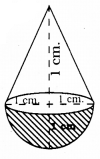∴ Volume of Total cube $$=\frac{2 \pi}{3}+\frac{\pi}{3} \mathrm{cm}^{3}$$
= πcm3

Question 2.
Rachel, an engineering student, was asked to make a model shaped like a cylinder with two cones attached at its two ends by using a thin aluminium sheet. The diameter of the model is 3 cm and its length is 12 cm. If each cone has a height of 2 cm, find the volume of air contained in the model that Rachel made. (Assume the outer and inner dimensions of the model to be nearly the same.)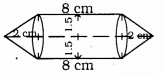solution:
Volume of cylinder, V = πr2h
= π × (1.5)2 × 8
= 18π cm3.
Volume of Cylinder V $$=\frac{1}{3} \pi r^{2} h$$
$$=\frac{1}{3} \pi \times(1.5)^{2} \times 2$$
$$=\frac{3}{2} \pi \mathrm{cm}^{3}$$
∴ The volume of air contained in the model that Rachel made.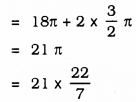= 66 cm3.

Question 3.
A Gulab Jamun contains sugar syrup upto about 30% of its volume. Find approximately how much syrup would be found in 45 gulab jamuns, each shaped like a cylinder with two hemispherical ends with length 5 cm and diameter 2.8 cm. (see figure given aside).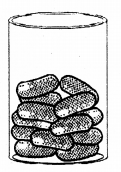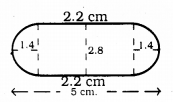Solution:
Length of cylindrical Gulab jamun,
l = 5 – (1.4 + 1.4) = 2.2 cm.
∴ Volume of Cylinder (1 Gulab Jamun), = Left hemisphere + Volume of Cylinder + Right hemisphere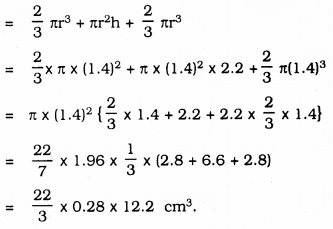∴ Total volume of such 45 Gulab jamun,
$$=45 \times \frac{22}{3} \times 0.28 \times 12.2$$
= 22 × 0.28 × 183
= 1127.28 cm3.
∴ Gulab Jamun contains sugar syrup upto about 30% of its volume.
∴ $$1127.28 \times \frac{30}{100}$$
= 338 cm3.

Question 4.
A pen stand made of wood is in the shape of a cuboid with four conical depressions to hold pens. The dimensions of the cuboid are 15 cm by 10 cm by 3.5 cm. The radius of each of the depressions is 0.5 cm and the depth is 1.4 cm. Find the volume of wood in the entire stand (see
Figure)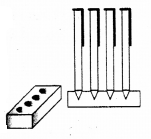Solution:
Radius of conical depression, r = 0.5 cm.
(Depth) height, h = 1.4 cm.
Volume of 1 depression which is conical, $$=\frac{1}{3} \pi r^{2} h$$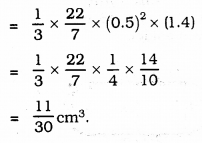Volume of such 4 depressions
$$=4 \times \frac{11}{30} \mathrm{cm}^{3}$$
$$=\frac{44}{30} \mathrm{cm}^{3}$$
∴ Total volume of wooden pen-stand, $$=(15 \times 10 \times 3.5)-\frac{44}{30}$$
= 525 – 1.47
= 523.53 cm3

Question 5
A vessel is in the form of an inverted cone. Its height is 8 cm and the radius of its top, which is open, is 5 cm. It is filled with water up to the brim. When lead shots, each of which is a sphere of radius 0.5 cm are dropped into the vessel, one-fourth of the water flows out. Find the number of lead shots dropped in the vessel.
Solution:
Volume of water in a Cone,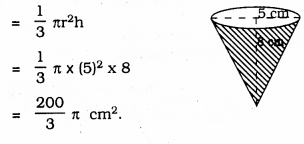Volume of lead shot $$=\frac{4}{3} \pi r^{3}$$
$$=\frac{4}{3} \pi \times(0.5)^{3}$$
$$=\frac{\pi}{6} \mathrm{cm}^{3}$$
Let the number of lead balls kept in vessel $$\frac{1}{4}$$ of the water flows out,
$$\Rightarrow n \times \frac{\pi}{6}=\frac{1}{4} \times \frac{200 \pi}{3}$$
$$\Rightarrow n \times \frac{\pi}{6}=\frac{100}{6} \pi$$
∴ n = 100
∴ Number of lead balls =100.

Question 6.
A solid iron pole consists of a cylinder of height 220 cm and base diameter 24 cm, which is surmounted by another cylinder of height 60 cm and radius 8 cm. (Find the mass of the pole, given that 1 cm3 of iron has approximately 8g mass. (Use π = 3.14).
Solution:
Height of solid iron pole, h = 220 cm.
Base diameter of pole, d = 24 cm.
∴ Radius, r = 12 cm.
Height of cylinder, h = 60 cm.
Radius of cylinder, r= 8 cm.
Mass of the pole = ?
Volume of 1st Cylinder, V = πr2h
= π × (12)2 × 220
Volume of 2nd cylinder, V = πr2h
= 77 × (8)2 × 60
∴ Total Volume = π × (12)2 × 220 + π × (8)2 × 60
= {144 × 220 + 64 × 60}π
= 35520π
= 111532.8 cm3.
Mass of iron about 1 ccm is 8 gm.
∴ Mass of iron 111532.8 ……. ??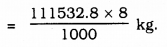= 111.5328 × 8 kg.
= 892.26 kg.

Question 7.
A solid consisting of a right circular cone of height 120 cm and radius 60 cm standing on a hemisphere of radius 60 cm is placed upright in a right circular cylinder full of water such that it touches the bottom. Find the volume of water left in the cylinder, if the radius of the cylinder is 60 cm. and its height is 180 cm.
Solution:
(i) Radius of cylinder GHEF, r = 60 cm.
Height, h = 120 + 60
= 180 cm.Volume of cylinderical vessel $$=\pi r^{2} h$$
= π × (60)2 × 180
(ii) Volume of hemisphere + Volume of cone(iii) Quality of water remained in cylinder.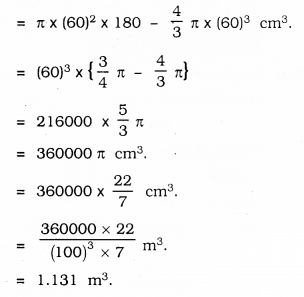Question 8.
A spherical glass vessel has a cylindrical neck 8 cm long, 2 cm in diameter; the diameter of the spherical part is 8.5 cm. By measuring the amount of water it holds, a child finds its volume to be 345 cm3. Check whether she is correct, taking the above as the inside measurements and n = 3.14.
Solution:
Diameter of spherical glass vessel = 8.5 cm.
∴ r = 4.25 cm.
Length of cylindrical neck = 8 cm.
Diameter = 2 cm.
∴ Radius, r = 1 cm.
Volume of water she found = 345 cm3.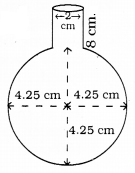(i) Volume of Cylinder neck, V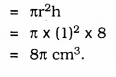(ii) Volume of cylindrical vessel $$=\frac{4}{3} \pi r^{3}$$
$$=\frac{4}{3} \times \pi \times(4.25)^{3}$$
∴ Total volume of water, V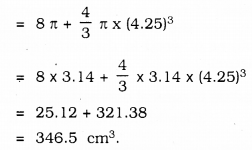∴ Her answer is not correct.

We hope the given KSEEB SSLC Class 10 Maths Solutions Chapter 15 Surface Areas and Volumes Ex 15.2 will help you. If you have any query regarding Karnataka SSLC Class 10 Maths Solutions Chapter 15 Surface Areas and Volumes Exercise 15.2, drop a comment below and we will get back to you at the earliest.

error: Content is protected !!